# Hyperbola Parts

 Given a hyperbola only, construct its transverse and conjugate axes, center, vertices, foci, directrices, and asymptotes.
 A hyperbola has two separate continuous parts. Begin by constructing a chord across one of these parts. Construct the midpoint of the chord.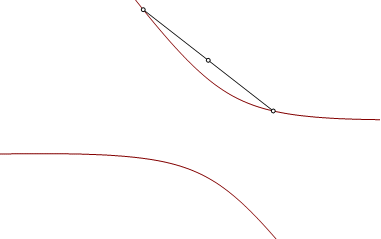Construct a parallel chord. This second chord may be on either part of the curve. Construct the midpoint of the second chord.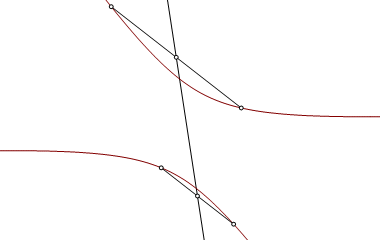The line joining the midpoints is an axis of oblique symmetry. It intersects the curve at two points. Let O be the midpoint of the chord joining the two intersections. This is the center of the hyperbola.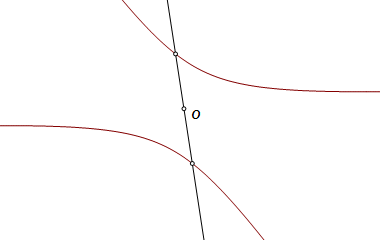Construct a circle centered on O and intersecting the hyperbola at four points. These four points are the vertices of a rectangle.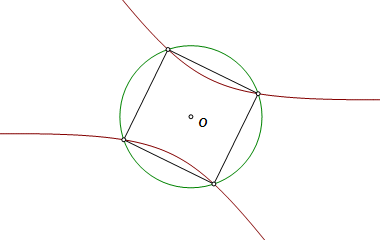The lines joining midpoints of opposite sides of the rectangle are axes of orthogonal symmetry. One of them intersects the hyperbola at two points. This is the transverse axis, and the intersections, V1 and V2, are the vertices. The other axis is the conjugate axis.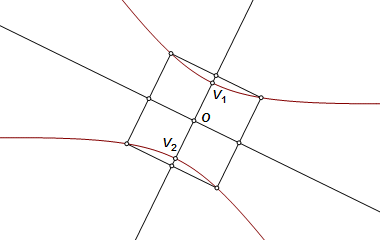Construct an arbitrary point P on the curve, and project it orthogonally onto the tansverse axis at point Q. The ratio PQ2 : (V1Q)(V2Q) is constant over all points P on the curve. Construct the circle centered on Q and dividing V1V2 harmonically, cutting it internally at point R.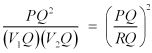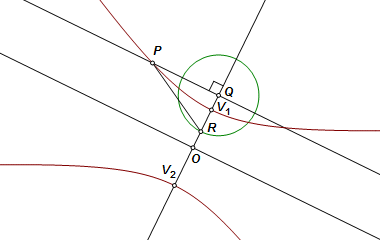The constant above is also the square of the ratio of the ordinate and abscissa measures from the center to any point on an asymptote. Therefore, line RP is parallel to one of the asymptotes. Construct a parallel line through the center. Reflect this asymptote on either of the axes to get the other asymptote.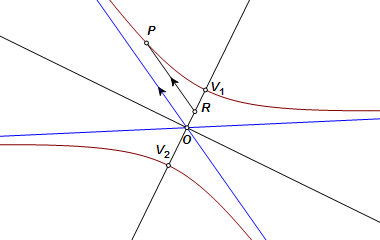Through vertex V1 construct the line perpendicular to the transverse axis. Let it intersect one of the asymptotes at point S. The ratio OS : OV1 must equal the ratio of the focus distance to the vertex distance. The circle centered on O and through S is used to construct the foci. Incidentally, this ratio is also the eccentricity of the hyperbola.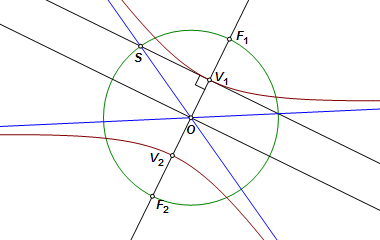Construct the circle on the diameter of the hypebola. The directrices are the polars of the foci with respect to this circle. They are also concurrent with the circle and the asymptotes, a fact which aids the construction.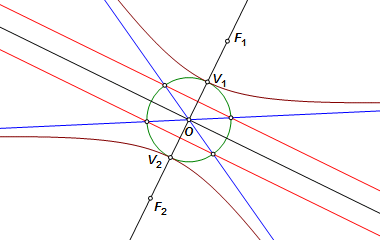The completed construction.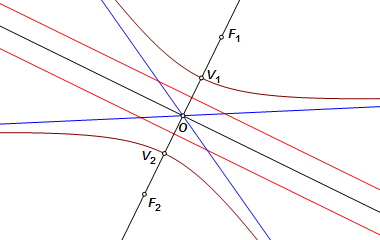Back to Conic Constructions Back to Whistler Alley Mathematics Last update: August 11, 2013 ... Paul Kunkel whistling@whistleralley.com For email to reach me, the word geometry must appear in the body of the message.